﻿ A Markov Chain Representation of Human Reasoning and Scientific ThinkingPublications are Open
Access in this journal
Article Versions
Export Article
• Normal Style
• MLA Style
• APA Style
• Chicago Style
Research Article
Open Access Peer-reviewed

### A Markov Chain Representation of Human Reasoning and Scientific Thinking

Michael Gr. Voskoglou
American Journal of Applied Mathematics and Statistics. 2020, 8(2), 52-57. DOI: 10.12691/ajams-8-2-3
Received May 29, 2020; Revised June 30, 2020; Accepted July 09, 2020

### Abstract

Reasoning is the most important human brain operation that leads to creative methodologies, algorithms and deductions giving way to sustainable research and development. Since the basis of reasoning in the Artificial Intelligence’s expert systems is human notions and concepts, the success of those systems depends upon the correspondence between human reasoning and their formalization. The paper at hands studies human reasoning in general and scientific thinking in particular with the help of the theory of finite Markov chains. Examples are also presented illustrating the applicability of the developed Markov chain models to real life situations.

### 1. Introduction

Artificial Intelligence (AI) is the branch of Computer Science focusing on the theory and practice of creating “smart machines” that are able to think, hear, talk, walk and even feel, like the humans do 1, 2. The term AI was coined by J. McCarthy in 1956, when he held in Dartmouth College, USA the first academic conference on the subject 3. However, the effort to understand if machines can truly think began much earlier, even before the Alan Turing’s abstract “learning machine” invention in 1936, which has been proven capable of simulating the logic of any computer’s algorithm 4.

AI as a synthesis of ideas from mathematics, engineering, technology and science (see Figure 1, retrieved from 5) has rapidly developed in the last 50-60 years creating a situation that has the potential to generate enormous benefits to the human society. The spectrum of AI covers many research areas and technologies, like knowledge engineering, data mining, reasoning methodologies, cognitive computing and modeling, machine learning, natural language processing and understanding, artificial planning and scheduling, vision and multimedia systems, intelligent tutoring and learning systems, etc.

• Figure 1. The interdisciplinary science of AI: A graphical approach

Since the basis of reasoning in the AI’s expert systems is human notions and concepts, the success of those systems depends upon the correspondence between human reasoning and their formalization. Thus, the study of human reasoning appears as a powerful theoretical framework for the development of the expert systems.

Human reasoning is characterized by inaccuracies and uncertainties, which stem from the nature of humans and the world. In fact, none of our senses and observation instruments allows us to reach an absolute precision in a world which is based on the principle of continuity, as opposed to discrete values.

Probability theory offers ideal conditions for tackling the cases of uncertainty which are due to randomness. Edwin T. Jaynes (1922-1998), Professor of Physics at the University of Washington, was one of the first suggesting in the middle of 1990’s that probability theory is a generalization of the bivalent logic reducing to it in the special case where our hypotheses are considered to be either absolutely true or absolutely false 6. Many eminent scientists have been inspired by the ideas of Jaynes. Among those is the Fields medalist David Mumford, who believes that Probability theory and statistical inference are emerging now as a better foundation of scientific models and even as essential ingredients for the foundation of mathematics 7.

In particular, the Markov Chain (MC) theory is a smart combination of Probability and Linear Algebra that is used in problems of AI to model something that is in discrete states, but it is not fully understood how it is evolved 8.

The paper at hands aims at representing human reasoning in general and scientific thinking in particular with the help of the theory of MCs. The rest of the paper is formulated as follows:

In Section 2 the mechanisms of human reasoning and of scientific thinking are described. In Sections 3 and 4 the MC models for human reasoning and scientific thinking respectively are developed accompanied by examples illustrating their applicability in real life applications. The article closes with the final conclusions and some hints for future research, presented in Section 5.

### 2. Human Reasoning and Scientific Thinking

Reasoning is the most important human brain operation that leads to creative methodologies, algorithms and deductions giving way to sustainable research and development.

Having a problem at hand, once the collection of the related linguistic information from the environment is completed, the human inquiry expands the field of understanding along different directions. In the mind each item concerning the phenomenon under investigation is labeled by a word or a set of words (statements, propositions). In this way the natural, environmental or engineering reality is divided into fragments and categories, which are fundamental ingredients in classification, analysis and deduction of conclusions.

In the next step, for any external object human beings try to imagine its properties in their minds. This gives them the power of initializing their individual thinking domain with whole freedom in any direction. Imagination includes the setting up of a suitable hypothesis or a set of logical rules for the problem at hand.

In the step of visualization humans use a variety of representations to defend their hypotheses including sketches, diagrams, charts, figures, etc. In particular, the geometric configuration of the objects appearing through imagination is the most common among those representations. In fact, after an object comes into existence vaguely in mind, it is necessary to know its shape, which is related to geometry. It is essential that the geometric configuration of the phenomenon must be visualized in mind in some way, even though it may be a simplification under a set of assumptions. This is the reason why geometry was developed and recognized by philosophers and scientists much earlier than any other scientific tools.

On the basis of their hypotheses humans generate relevant ideas. The ideas begin to crystallize and they are expressed verbally by a native language to other individuals to get their criticisms, comments, suggestions and support for the betterment of the mental thinking. It is emphasized that, whatever are the means of reasoning, the arguments are expressed verbally prior to any symbolic abstractions.

In conclusion the main steps of the process of human reasoning involve ( 9, Section 4]):

Ÿ S1 = understanding

Ÿ S2 = perceptions (feelings through imagination)

Ÿ S3 = sketches (design through visualization)

Ÿ S4= ideas generation.

The perceptions part is very important, because it provides complete freedom of thinking without expressing it to others, who can restrict the activity. The subjectivity is the main characteristic of the above part, but as one enters the sketch domain the subjectivities decrease and at the final stage, since the ideas are exposed to other individuals, the objectivity overrules becoming at least logical.

Note that the first three of those steps are continuous, since the completion of each one of them usually needs some time characterized by transitions between hierarchically neighboring steps. For example, being at the step of perceptions (S2) the individual may return to S1 for a better understanding of the corresponding problem, being at S3 and having difficulties to sketch properly his/her perceptions, he/she may return to S2 to clarify them better, etc. Therefore, a graphical representation of the reasoning process is that shown in Figure 2.

• Figure 2. A graphical representation of the process of human reasoning

Scientific thinking is obviously a subclass of human reasoning. The process of scientific thinking is graphically represented in Figure 3, retrieved from 10. In this Figure a1, a2, …., an are observations of the real world that have been transformed, by induction, to the theory T1. Theory T1 was verified by deduction and additional deductive inferences K1, K2,…, Ks were obtained. Next, a new series of observations b1, b2,…, bm follow. If some of those observations are not compatible to the laws of theory T1, a new theory T2 is formed by induction (intuitively) to replace/extend T1. The deductive verification of T2 is based on axioms partially or even completely different to those of theory T1 and new deductive inferences L1, L2,…, Lt follow. The same process could be repeated (observations c1, c2,…, ck , theory T3, inferences M1, M2,…, Mp, etc.) one or more times. In each case the new theory extends or rejects the previous one approaching more and more the absolute truth.

• Figure 3. The scientific method

This procedure is known as the scientific method. The term was introduced in the 19th century, when significant terminologies appeared establishing clear boundaries between science and non science. However, the scientific method characterizes the development of science since at least the 17th century. Aristotle (384-322 BC) is recognized as the inventor of the scientific method due to his refined analysis of the logical implications contained in demonstrative discourse. The first book in the history of human civilization written on the basis of the principles of the scientific method is, according to the existing witnesses, the “Elements” of Euclid (365-300 BC) addressing the axiomatic foundation of Geometry.

The scientific method is highly based on the Trial and Error procedure, a term introduced by C. Lloyd Morgan (1852-1936) 11. This procedure is characterized by repeated attempts, which are continued until success or until the subject stops trying. In other words, one could say that scientific thinking is a synthesis of repeated attempts of human reasoning. Therefore, under the light of Figure 2, the process of scientific thinking can be graphically represented as it is shown in Figure 4.

• Figure 4. The process of scientific thinking

In fact, when the verification of theory T1 is integrated in S4, the new observations b1, b2,…, bm that follow may be or not be compatible to the laws of theory T1. In the first case the process of scientific thinking remains to S4, whereas in the second case a new attempt starts for developing theory T2. This means that the process returns to step S1 and the same circle is repeated again, and so on.

Sir Karl Raimund Popper (1902-1994), one of the 20th century’s most influential philosophers of science, proposed the principle of falsification to distinguish between science and pseudo-science. According to this principle a proposition can be characterized as scientific, if, and only if, it includes all the necessary criteria for its control, i.e. if, and only if, it could be falsified 12. Critiques on the principle of Popper report that he used it to decrease the importance of induction for the scientific method 13.

It is of worth noticing that the error of inductive reasoning is transferred to deduction through its premises, which are always obtained by induction ( 10, Section 3). Therefore, the scientific error in its final form is actually a deductive and not an inductive error! However, many philosophers consider deduction as being an infallible method due to the following two reasons:

Ÿ Deduction is always a consistent method, i.e. the truth of its premises guarantees the truth of the deductive inference.

Ÿ The existing theories (used as premises to deduction) are considered as being always true, which leads frequently to surprises.

The Scottish philosopher David Hume (1711-1776) argued that, with deductive reasoning only, humans would be starving. Although this could not happen nowadays due to our past knowledge obtained with the help of induction, without inductive reasoning no further scientific progress could be achieved!

Thus, it is crucial to find a better way than the Poppers’ falsification principle, to distinguish science from pseudo-science by assigning a degree of truth to each possible case. This gave us in an earlier work ( 10, Section 5) the impulsion to apply principles of fuzzy logic and Bayesian probabilities for this purpose. Here, as we have already statedf in our Introduction, we are going to study human reasoning and scientific thinking with the help of the theory of finite MC’s.

### 3. The Markov Chain Model for Human Reasoning

The basic principles of the MC theory were introduced in 1907 by the Russian mathematician A. Markov (1856-1922) through his efforts of coding literal texts. Since then, the corresponding theory has rapidly developed and its importance for probabilistic reasoning has been recognized to the natural, social and applied sciences 14, 15, 16, 17.

A MC is defined as a stochastic process that moves in a sequence of steps through a set of states and has only a one-step memory. This means that, being in a certain state at a certain step, the probability of entering another (or the same) state in the next step (transition probability) depends only on the state occupied in previous step and not in earlier steps (Markov property).

However, since the application of the MC theory enables one to make accurate forecasts for the evolution of a great number of random phenomena, many authors, in their effort to cover as many as possible such phenomena using MC’s, have weakened the Markov property by accepting that, although the transition probability from one state to another depends mainly on the state occupied in the previous step, need not be completely independent from the states occupied in previous steps (e.g. see 18, Chapter IV, Section 12, 17 Chapter 2, pp. 33-34, etc.).

When the set of states of a MC is finite, the chain is called a finite MC. For basic facts about a finite MC we refer to Chapters 2 and 3 of the book 17, while for more detailed proofs the reader may look the classical on the subject book 14 or any other of the many modern books that are available in the literature (e.g. 15, 16, etc.).

Here, we introduce a finite MC on the steps Si, i = 1, 2, 3, 4, of human reasoning, as they have been described in the previous Section. Consider the probability vector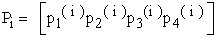where pk(i) denotes the probability of the MC to be in state Sk at the i-th step, k=1, 2, 3, 4 and i = 0, 1, 2,.... . It is well known then that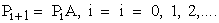(1)

Equation (1) enables one to make short run forecasts for the evolution of the reasoning process (see Example 1). Since in our case S1 is always the starting state of the MC, one finds that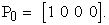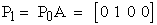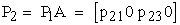(2)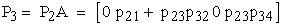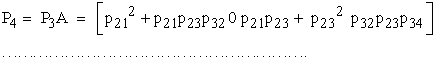and so on.

Observe also that, when the chain reaches the state S4 it is impossible to leave it. In other words, S4 is an absorbing state of the MC. Further, since it is possible from any state to reach the absorbing state S4, not necessarily in one step (see Figure 2), our MC is an absorbing MC (AMC) having S4 as its unique absorbing state.

Denote by pij the transition probability from state Si to Sj, i, j = 1, 2, 3, 4. Then, with the help of Figure 2, one finds that the transition matrix of the MC is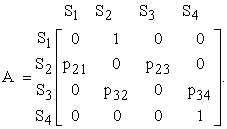with p21+p23 = p32+p34 = 1 (probability of the certain event).

Applying standard results of the theory of the AMC’s, we bring the transition matrix A to its standard form A* by listing its absorbing state first and we make a partition of A* to sub-matrices as follows: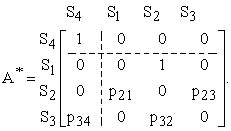Therefore, the transition matrix of the non absorbing states is equal to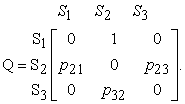Denote by I3 the 3X3 unitary matrix. Then the determinant D(I3-Q)=1-p23p32-p21=p23-p23p32= =p23(1-p32)=p23p34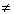0, therefore I3-Q is an invertible matrix. The fundamental matrix of the AMC is calculated by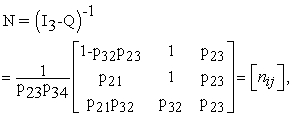with i, j = 1, 2, 3.

It is well known that the element nij of N is equal to the mean number of times in state Sj before the absorption, when the MC starts from the state Si, where Si and Sj are non absorbing states. Therefore, since in our case S1 is always the starting state, the mean number of steps taken before the absorption is equal to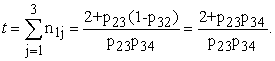(3)

The minimal value of t is equal to 3, corresponding to the case where we have no backward transitions between neighboring states (Figure 2). It becomes evident that the greater is the value of t, the more the difficulties during the reasoning process. Another indication about those difficulties is of course the total time spent by the individual during the reasoning process.

The following example illustrates the applicability of the previous MC model to real conditions.

Example 1: We want to construct a channel to run water by folding the two edges of a rectangular metallic leaf having sides of length 20 cm and 32 cm, in such a way that they will be perpendicular to the other parts of the leaf. Assuming that the flow of the water is constant, how we can run the maximum possible quantity of the water?

Analysis of the problem

Imagination: The basic thing to realize is that the quantity of water to run through the channel depends on the area of the vertical cut of the channel.

Visualization (geometric configuration): Folding the two edges of the metallic leaf by length x across its longer side the vertical cut of the constructed channel is an orthogonal with sides x and 32-2x (Figure 5).

• Figure 5. The vertical cut of the channel

Ideas generation: The area of the orthogonal has to be maximized. This idea leads to the following mathematical manipulation: The area is equal to E(x)=x(32-2x)=32x-2x2. Taking the derivative E΄(x) the equation E΄(x)=32-4x=0 gives that x=8cm. But E΄΄(x)=- 4<0, therefore E(8)=128 cm2 is the maximum possible quantity of water to run through the channel.

Assume now that at the first glance the solver did not understand well the statement of the problem and that he tried to construct the channel by curving the metallic leaf. As a result, the vertical cut of the leaf turned to be a parabola. The solver realized the complexity of the problem in that case and, by studying more carefully the problem’s statement, he understood the proper way of constructing the channel. However, in his next effort he folded the shorter side of the leaf and he found E(x)=x(20-2x)=20x-2x2. In that case the equation E΄(x)=20-4x=0 gives that x=5cm and therefore E(5)= 50 cm2. Finally, the solver decided to fold also the longer side of the leaf and by comparing the two outcomes he found the correct solution.

Observe now with the help of Figure 2 that, when the MC reaches S2 for first time, it returns to S1. However, when it comes back from S1 to S2 for second time, then it proceeds to S3, wherefrom it returns to S2 to proceed again to S3. Therefore, we have that p21=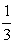and p23=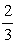. In the same way it is straightforward to check that p32= p34=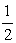. Replacing those values to equation (3) one finds that t = 7 steps.

Also, the third of equations (2) gives that P3=[00]. Therefore, the probability to be in state S2 at the fourth step of the process is equal to p2(3) =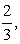and so on.

### 4. The Markov Chain Model for Scientific Thinking

Here, we introduce a finite MC on the steps Si, i = 1, 2, 3, 4, of scientific thinking, as they have been described in Section 2. With the help of Figure 4 it is straightforward to check that the transition matrix of this chain is equal to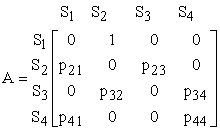Further, from Figure 4 it becomes evident that in this MC there is no absorbing state. Also it is not possible to go between any two states; e.g. being in state S4 it is not always possible to go to state S1 and through it to any other state. It is recalled that a MC is said to be an ergodic MC (EMC), if from any state we can go to any other state, not necessarily in one step. Therefore, the constructed chain is neither an AMC, nor an EMC.

Note that, as the number of steps of an EMC tends to infinity (long run), the chain tends to an equilibrium situation, in which the probability vector Pk takes a constant price P = [p1 p2 …. pn], with p1+p2+p3+p4 = 1. P is called the limiting probability vector of the EMC. Therefore, equation (1) gives in this case that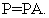(4)

The entries of P express the probabilities of the EMC to be in each of its states in the long run, i.e. the importance (gravity) of each state of the EMC.

In other words, only in case of an EMC one can make long run forecasts for the evolution of the corresponding situation. Consequently, one cannot make long run forecasts for the evolution of the scientific theories. The following examples from the history of science illustrate the importance of this remark.

Example 2: The geocenrtic theory (Almagest) of Ptolemy of Alexandria (100-170), being able to predict satisfactorily the movements of the planets and the moon, was considered to be true for centuries. However, it was finally proved to be wrong and has been replaced by the heliocentric theory of Copernicus (1473-1543). The Copernicus’ theory was supported and enhanced a hundred years later by the observations/studies of Kepler and Galileo. But, although the idea of the earth and the other planets rotating around the sun has its roots at least to the time of the ancient Greek astronomer Aristarchus of Samos (310-230 B.C.), the heliocentric theory faced many obstacles for a long period of time, especially from the part of the church, before its final justification 19.

Example 3: Euclid created the theoretical foundation of the traditional Geometry on the basis of 10 axioms, which were used to prove all the other known on that time geometric propositions and theorems. The fifth of those axioms, stated in its present form by Proclus (412-485), says that from a point outside a given straight line only one parallel can be drawn to this line. However, this axiom does not have the plainness of the rest of the Euclid’s axioms. This gave during the centuries to many mathematicians the impulsion to try to prove the fifth axiom with the help of the other Euclid’s axioms.

One of the latest among those mathematicians was the Russian Lobachevsky who, when he failed to do so, he decided to investigate what happens if the fifth axiom does not hold. Thus, replacing (on a theoretical basis) that axiom by the statement that AT LEAST TWO parallels can be drawn from the given point to the given line, he created the hyperbolic geometry, which is developed on a hyperbolic paraboloid’s (saddle’s) surface. The Riemann’s elliptic geometry on the surface of a sphere followed, which is based on the assumption that NO PARALLEL can be drawn from the given point to the given straight line. The elliptic geometry for small distances on the earth reduces to the traditional Euclidean geometry.

Example 4: Approximately 50 years after the development of the non Euclidean geometries Einstein expressed his strong belief that the Newton’s calculation of the gravitational force F between two masses m1 and m2 by the formula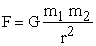where G stands for the gravitational constant, was not correct for the existing in the Universe (outside the earth) strong gravitational forces (general relativity theory). This new approach was based on the fact that, according to Einstein’s special theory of relativity (1905) the distance (r) and the time (t) are changing in a different way with respect to a motionless and to a moving observer.

To support his argument Einstein introduced the concept of the 4-dimensional time-space and after a series of intensive efforts (1908-1915) he finally managed to prove that the geometry of this space is non Euclidean! For example, the non Euclidean divergence of the radius r of a sphere of total surface S and mass m is, according to Einstein’s theory, is equal to r -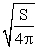=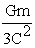.

The non Euclidean form of the time-space is physically explained by its distortion created by the presence of mass or of an equivalent amount of energy. This appears analogous to the distortion created by a ball of bowling on the level of a trampoline.

Einstein’s theory was experimentally verified by the irregularity of the Hermes’ orbit around the sun although on that time many scientists argued that this irregularity was due to the existence of an unknown planet near Hermes or an unknown satellite of Hermes or a group of asteroids near the planet. However, the magnitude of the divergence of the light’s journey, which was calculated during the eclipse of the sun on May 29, 1919, was the definite evidence for the soundness of Einstein’s theory. In fact, the eclipse let some stars, which normally should be behind the sun, to appear besides it on the sky 20.

### 5. Conclusion

The following conclusions can be drawn from the discussion performed in this work:

Ÿ The process of human reasoning can be studied by developing an AMC on its steps.

Ÿ Scientific thinking is a synthesis of repeated attempts of human reasoning, which is based on a series of observations of the real world. Therefore, the process of scientific thinking can be also represented by a MC, which however is neither an AMC nor an EMC. This proves mathematically that no long run forecasts can be made for the evolution of the scientific theories.

Ÿ Examples from the history of science were presented illustrating the previous conclusion.

Several other applications of AMCs and EMCs to topics like education, decision-making, case-based reasoning, management, economics, etc. have been presented in earlier works of the author; e.g. see 17, Chapters 2 and 3 and the relative references contained in it, 21, 22, etc. We plan to focus a great part of our future research too on this interesting subject.

### References

  Mitchell, M. Artificial Intelligence: A Guide for Thinking Humans; Parrar, Straus and Gtraux: New York, NY, USA, 2019. In article  Kastranis, A. Artificial Intelligence for People and Business; O’ Reily Media Inc.: Sebastopol, CA, USA, 2019. In article  Moor, J., “The Dartmouth college artificial intelligence conference: The next fifty years”, AI Mag., 27, 2006, 87-91. In article  Hodges, A. Alan Turing: The Enigma (The Centenary Edition); Princeton University Press: Princeton, NJ, USA, 2012. In article View Article  Voskoglou, M.Gr. & Salem A.-B.M., “Benefits and Limitations of the Artificial with Respect to the Traditional Learning of Mathematics”, Mathematics, 8, 2020, 611. In article View Article  Janes, E.T., Probability Theory: The Logic of Science, Cambridge University Press, UK, 8th Printing, 2011 (first published, 2003). In article  Mumford, D., “The Dawing of the Age of Stochasticity”, in V. Amoid, M. Atiyah, P. Laxand & B. Mazur (Eds.), Mathematics: Frontiers and Perspectives, AMS, 2000, 197-218. In article  Domingos, P. & Lowd, D., Markov Logic: An Interface Layer for Artificial Intelligence, Morgan & Claypool Publishers, Williston, VT, USA, 2009. In article View Article  Sen, Z., “Fuzzy philosophy of science and education”, Turkish Journal of Fuzzy Systems, 2 (2), 2011, 77-98. In article  Athanassopoulos, E. & Voskoglou, M.Gr., “A Philosophical Treatise on the Connection of Scientific Reasoning with Fuzzy Logic”, Mathematics, Vol. 8, 2020, 875. In article View Article  Thrope, W.H., The origins and rise of ethology: The science of the natural behavior of animals, Praeger, London-NY, 1979. In article  Popper, K. R., The Logic of Scientific Discovery, Routledge, Oxon, UK, 2002 (Edition in German, 1934, translated in English, 1959). In article  Ladyman, J., Understanding the Philosophy of Science, Routledge, Oxon, UK, 2002. In article  Kemeny, J.G. & Snell, J.L., Finite Markov Chains, Springer-Verlag, New York, 1976. In article  Zucchini, I.L., MacDonald, I.L. &. Langrock, R., Hidden Markov Models for Time Series, Second Edition, CRC Press, NY, 2016. In article View Article  Davis, M.H.A., Markov Models and Optimization, Create Space Independent Publishing Platform (Amazon), Columbia, SC., USA, 2017. In article  Voskoglou, M.Gr., Finite Markov Chain and Fuzzy Logic Assessment Models: Emerging Research and Opportunities, Create Space Independent Publishing Platform (Amazon), Columbia, SC, USA, 2017. In article  Kemeny, J.G., Schleifer, A.J. & Snell, J.L., Finite Mathematics with Business Applications, Prentice Hall, London, 1962. In article  Gingerich, O., The Eye of the Heaven - Ptolemy, Copernicus, Kepler, American Institute of Physics, NY, 1993. In article  Singh, S., Bing Bang - The Origin of the Universe, Harper Perennian Publishers, NY, 2005. In article  Voskoglou, M. Gr., “An Application of Ergodic Markov Chains to the process of teaching mathematics”, American Journal of Applied Mathematics and Statistics, 7(5), 2019, 187-190. In article  Voskoglou, M. Gr., “A Markov Chain Application on the Levels of the Bloom’s Taxonomy of Learning Objectives”, American Journal of Educational Research, 7(3), 2019, 294-298. In article View ArticleThis work is licensed under a Creative Commons Attribution 4.0 International License. To view a copy of this license, visit http://creativecommons.org/licenses/by/4.0/